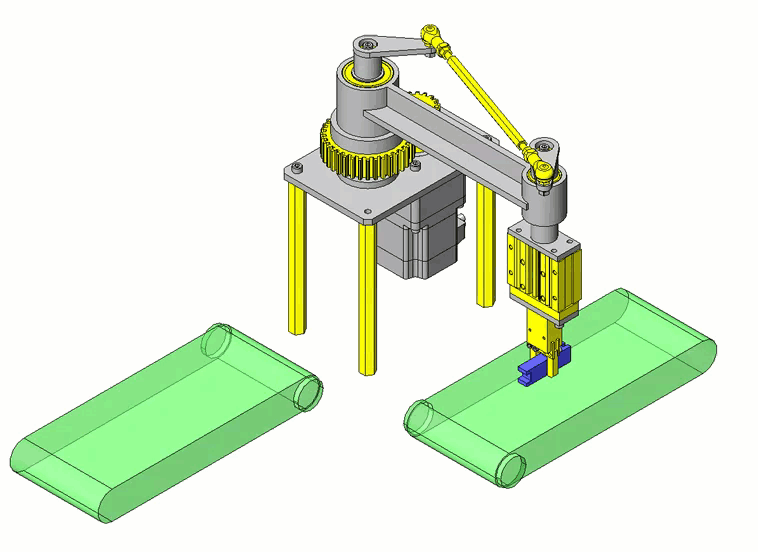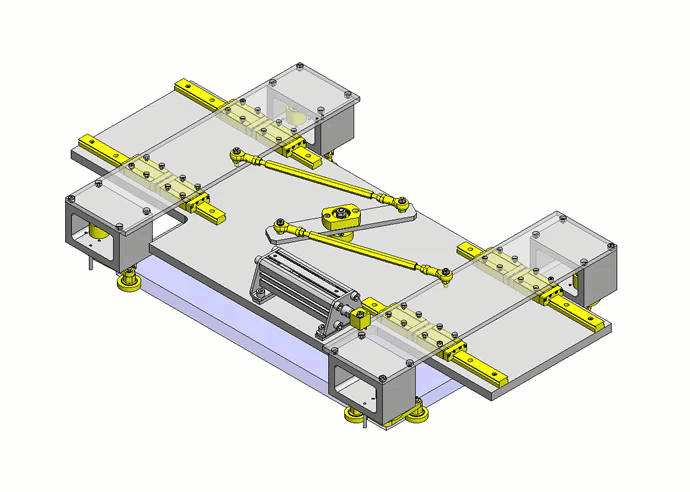# Give Your Design a Boost: Boost Mechanisms

A mechanism designed to generate a large force from a small force is called a boost mechanism. Mechanical components that constitute this boost mechanism include links, levers, screws, wedges, gears, and pulleys. For lightweight arms of robots, the link mechanism is frequently adopted for its boosting effect produced from the simple structure. Lifting up a heavy load using a lever is also an application example of this boost mechanism. In this case, you will be able to lift up a heavy load with a small force, but you will need to apply the force for a longer distance than when you carry the heavy load itself. Using a boost mechanism does not change the workload, the force required for moving an object multiplied by the travel distance.

Let’s take a look at the link mechanism consisting of four-bar linkages in [Fig.1] – a) and consider how the force vector is applied at Joint A.
[Fig.1] – b) is the schematic layout of a link mechanism generating a high degree of boosting effect.
[Fig.1] – c) is the schematic layout of a link mechanism generating little boosting effect.
Let’s compare their force vector components at Joint A.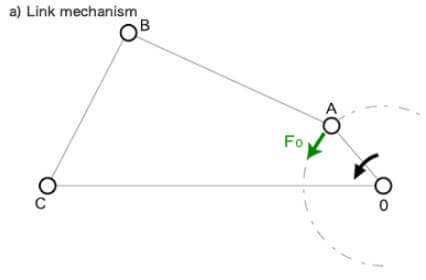In [Fig.1] – b), “Crank OA” and “Link AB” form an angle of approximately 180 degrees (close to a linear arrangement) whereas “Link AB” and “Lever BC” are almost perpendicular to each other. In this case, if  “Torque T” applies to “Driving Shaft OA” and Force F0 applies to the tangential direction of “Joint A”, Force F1 applied to “Link AB” on “Joint A” will be 2.7 times greater than the tangential force F’0 based on the vector components at “Joint A”.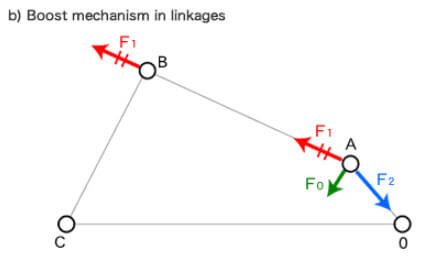In [Fig.1] – c) on the other hand, Force F’1 applied to “Link AB” on “Joint A” is only 1.1 times greater than the tangential force F’0.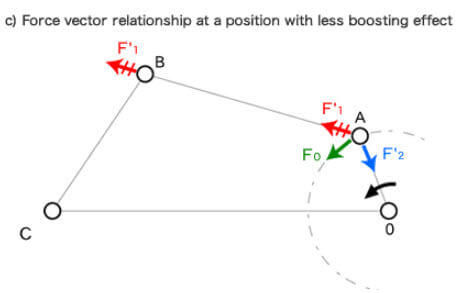As seen in this example, using the link mechanism to form the relationship shown in [Fig.1] – b), allows effective construction of the boost mechanism. In addition, when the link mechanism is applied to the boost mechanism, all the rotary couplers (in pairs) and the links must be designed with enforced strength.

Crank

The figure [a] below is a schematic view of the press machine. An eccentric disk is used for “Crank OA”. When the center of this disk (center of gravity) comes below the center of rotation O, the weight of this disk acts on the presswork.

In figure [b], the press machine [a] is expressed in the skeleton method. If we compare the vector components at “Joint A” in [b] and those at the pressing point “B”, we can see that a booster action can be obtained from them. Using an eccentric disk amplifies “Tangential Force F0” acting on “Joint A”. Then, the piston changes its force direction to vertical and forms “Press Force FP“. In this figure, “Press Force FP” is approximately 1.4 times greater than “Tangential Force F0“.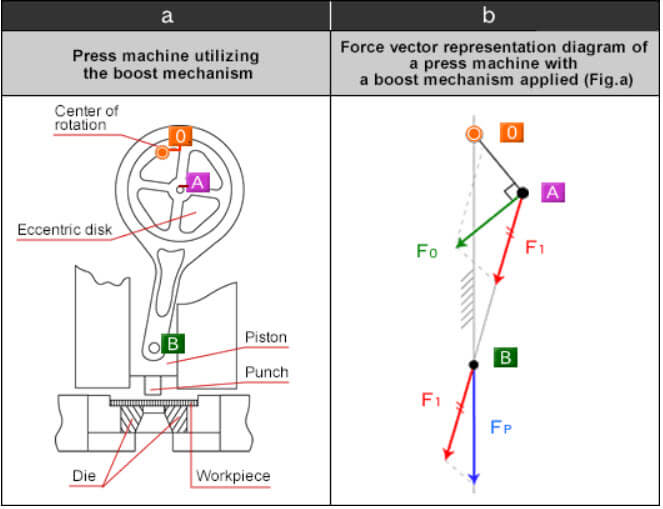Leverage

A bell-crank can be described as a bent version of a seesaw-type boost mechanism. The principle of the boost mechanism is the same, but because of the bent shape of the crank, the arm length is designed to be perpendicular to the direction of force applied, resulting in a different boosting effect ([Fig.2] – a)). A bell-crank is shaped the same as a nail puller used for carpentry work.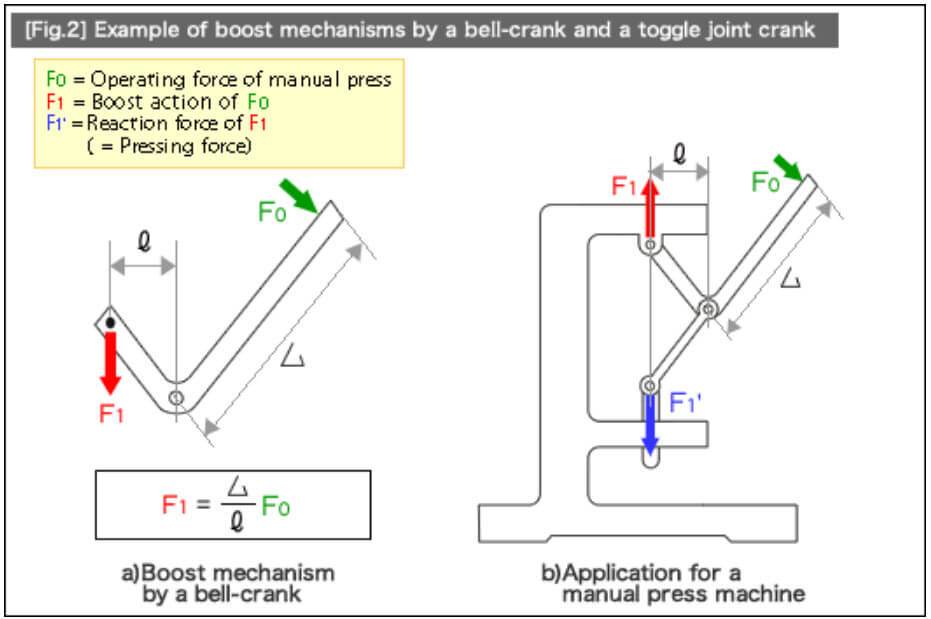If you combine a bell-crank with a toggle joint crank, you will be able to create a simple manual pressing machine shown in [Fig.2] – b). In this mechanism, the force applied to the bell-crank handle (F0) is amplified by the bell-crank’s boost mechanism in accordance with the arm length ratio and transformed into force (F1). The cabinet of the simple press machine supports this force. The toggle joint then diverts its reaction force toward the opposite direction, creating the pressing force (F1′). A “double-lever” is created by connecting levers utilized in the boost mechanism. The double-lever method not only delivers the boost action in the simple seesaw-type principle but also allows the direction of the force to be changed.

[Fig.1] – a) illustrates the boost mechanism for the double-lever method. The amplified force (F) is determined by the relationship between the arm length ratio and the equilibrium of forces.

[Fig.1] – b) is a simple fixture (tool) called a “block holder”, utilizing the double-lever method. This mechanism is often adopted for tools designed to carry heavy square-shaped items like bricks and blocks.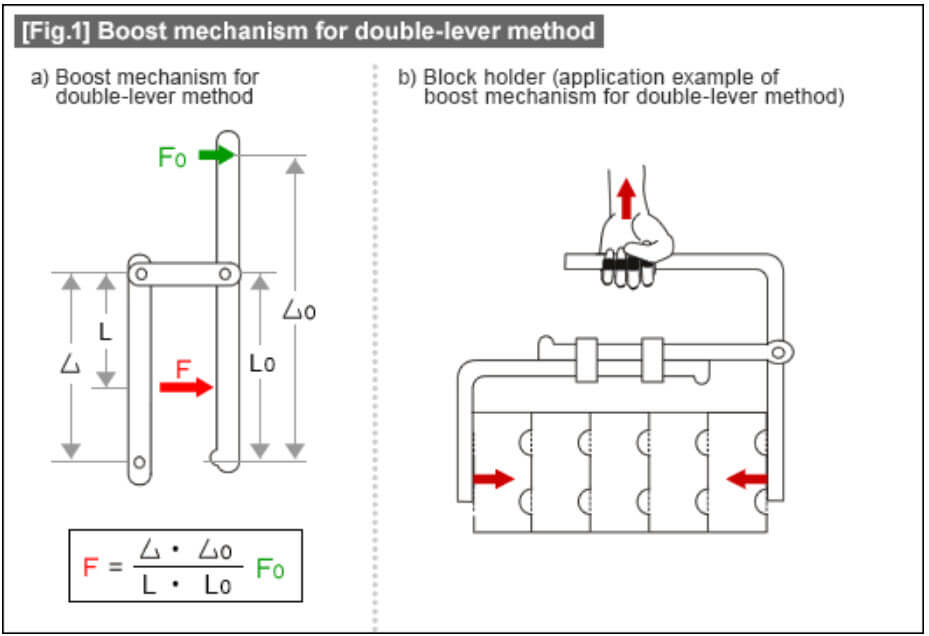Although the seesaw-type boost allows the operation of heavy objects with less of force, it is important to remember that the principle of the workload (Workload (Kgm) = Force (Kg) × Movement (m)) always applies. Less amount of force means more movement. If manpower is required for larger strokes or reducing a particular task cycle (such as moving or bending) does not make the entire task inefficient, it is possible to amplify the forces or movement based on this workload principle.

Application examples utilizing boost mechanisms

1. Workpiece (block) conveyance fixtures
2. Washing and Rinsing clamp
3. Conveyance clamp in heat-treating furnace
4. Manual press machine
5. Manual swaging machine
6. Manual crimping machine, thermocompression bonding machine
7. Flatness correction